# Microeconomic Theory

## Quiz 5 :Demand Relationships Among GoodsLooking for Economics Homework Help?

## Quiz 5 :Demand Relationships Among GoodsHeidi receives utility from two goods, goat's milk (m) and strudel (s), according to the utility function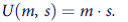a. Show that increases in the price of goat's milk will not affect the quantity of strudel Heidi buys; that is, show that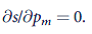b. Show also that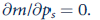c. Use the Slutsky equation and the symmetry of net substitution effects to prove that the income effects involved with the derivatives in parts (a) and (b) are identical. d. Prove part (c) explicitly using the Marshallian demand functions for m and s.
Free
Essay

Utility function is a mathematical function which represent the individual preference for the goods.
Substitution effect: It measures the change in demand due to change in relative prices keeping purchasing power constant.
Income effect: It measures the change in demand due to change in due to change in purchasing power keeping relative price constant.
a )
The given utility function is shown below:
Utility function: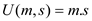Where, m denotes the goat's milk and s denote the strudel.
The condition of consumer equilibrium can be easily understood with the help of Lagrange multiplier.
Budget constraint can be for this problem is shown below: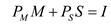By using above information, the following lagragian function is obtained which is shown below: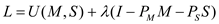Taking first order condition:By using above, the following result is obtained which is shown below: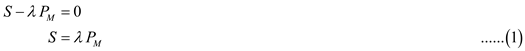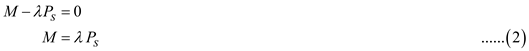Taking ratio of equation (1) and equation (2), the following result is obtained which is shown below:Now, substituting the value of M in the budget constraint, the following result is obtained which is shown below:Thus, from above value of s the following result is obtained which is shown below: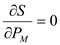This shows that increases in the price of goat's milk does not affected the quantity of strudel.
b )
The lagragian function for the given problem is shown below:Taking first order derivative, the following result is obtained which is shown below:By using above function, the following result is obtained which is shown below:Taking ratio of equation (1) and equation (2) the following result is obtained which is shown below:Substitute the M value in budget constraint:Thus, from above value of M the following result is obtained which is shown below: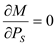The above result shows that increase in the price of strudel does not affect the quantity of goat's milk.
c )
In two goods case the income and substitution effects from the change in the price of one good on the demand for another good usually work in opposite directions. Its substitution effect is positive but its income effect is negative.
Slutsky equation:Where,
Uncompensated demand is represented by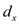,
Compensated demand or Hickson demand is represented by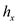.
To generatecreate the expenditure function and take the first derivative:The Hickson demand function is obtained by taking the derivative of expenditure function with respect to their prices as shown below:Now, taking the derivative of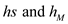with respect to their prices the following result is obtained which is shown below:Since,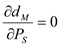and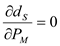It shows that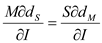Hence proved.
d )
The Marshallian demand function shows changes in the price of y do not affect x purchases.
That is,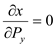Thus, by using this the following result is obtained which is shown below:Hence Proved.Hard Times Burt buys only rotgut whiskey and jelly donuts to sustain him. For Burt, rotgut whiskey is an inferior good thatexhibits Giffen's paradox, although rotgut whiskey and jelly donuts are Hicksian substitutes in the customary sense. Develop anintuitive explanation to suggest why an increase in the price of rotgut whiskey must cause fewer jelly donuts to be bought. Thatis, the goods must also be gross complements.
Free
Essay

Hard Times buys only rotgut whiskey and jelly donuts. Rought whiskey and jelly donuts are Hicksian substitute goods in customary sense. Since rotgut whiskey is an inferior good that exhibits giffen's paradox. Law of demand does not work in case of giffen good.
As we know that in case of giffen good, demand of the good is increased as price of the good increases and vice-versa. As price of rotgut whiskey increases, thus demand of rotgut whiskey is also increase. Hard Times' income remains the same. Now Hard Times expend more income to buy whiskey than that of earlier. Simultaneously he reduces his expenditure to buy jelly donuts. Therefore, an increase in the price of rotgut whiskey must cause fewer jelly donuts to be bought.Donald, a frugal graduate student, consumes only coffee ( c ) and buttered toast ( bt ). He buys these items at the university cafeteria and always uses two pats of butter for each piece of toast. Donald spends exactly half of his meager stipend on coffee and the other half on buttered toast. a. In this problem, buttered toast can be treated as a composite commodity. What is its price in terms of the prices of butter ( p b ) and toast ( p t )? b. Explain why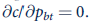. c. Is it also true here that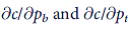are equal to 0?
Free
Essay

a.
Mr. D uses two pats of butter for each piece of toast.
The price of buttered toast can be shown in terms of price of butter and price of toast as given below: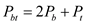Where,b.
Mr. D spends exactly half of his meager stipend on coffee (c) and the other half on buttered toast (bt).Differentiating value of bt and c with respect to each other prices as shown below: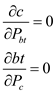is zero because demand of coffee is not a function of price of butter.
It means that change in price of butter toast does not affect the demand of coffee.
c.
Mr. D uses two pats of butter for each piece of toast.It is true that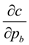and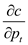are equal to zero as demand for coffee doesn't depend on the prices of toast and butter. Mr. D spends a fixed proportion of his income on the coffee. So price of toast and butter will not affect his demand for coffee.Ms. Sarah Traveler does not own a car and travels only by bus, train, or plane. Her utility function is given by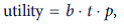where each letter stands for miles traveled by a specific mode. Suppose that the ratio of the price of train travel to that of bus travel ( p t / p b ) never changes. a. How might one define a composite commodity for ground transportation? b. Phrase Sarah's optimization problem as one of choosing between ground ( g ) and air ( p ) transportation. c. What are Sarah's demand functions for g and p ? d. Once Sarah decides how much to spend on g , how will she allocate those expenditures between b and t?
EssaySuppose that an individual consumes three goods, x 1 , x 2 , and x 3 , and that x 2 and x 3 are similar commodities (i.e., cheap and expensive restaurant meals) with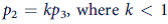-that is, the goods' prices have a constant relationship to one another. a. Show that x 2 and x 3 can be treated as a composite commodity. b. Suppose both x 2 and x 3 are subject to a transaction cost of t per unit (for some examples, see Problem 6.6). How will this transaction cost affect the price of x 2 relative to that of x 3 ? How will this effect vary with the value of t? c. Can you predict how an income-compensated increase in t will affect e x penditures on the composite commodity x 2 and x 3 ? Does the composite commodity theorem strictly apply to this case? d. How will an income-compensated increase in t affect how total spending on the composite commodity is allocated between x 2 and x 3 ?
EssayApply the results of Problem 6.5 to explain the following observations: a. It is difficult to find high-quality apples to buy in Washington State or good fresh oranges in Florida. b. People with significant babysitting expenses are more likely to have meals out at expensive (rather than cheap) restaurants than are those without such expenses. c. Individuals with a high value of time are more likely to fly the Concorde than those with a lower value of time. d. Individuals are more likely to search for bargains for expensive items than for cheap ones. Note: Observations (b) and (d) form the bases for perhaps the only two murder mysteries in which an economist solves the crime; see Marshall Jevons, Murder at the Margin and The Fatal Equilibrium. Reference: Problem 6.5 Suppose that an individual consumes three goods, x 1 , x 2 , and x 3 , and that x 2 and x 3 are similar commodities (i.e., cheap and expensive restaurant meals) with-that is, the goods' prices have a constant relationship to one another. a. Show that x 2 and x 3 can be treated as a composite commodity. b. Suppose both x 2 and x 3 are subject to a transaction cost of t per unit (for some examples, see Problem 6.6). How will this transaction cost affect the price of x 2 relative to that of x 3 ? How will this effect vary with the value of t? c. Can you predict how an income-compensated increase in t will affect e x penditures on the composite commodity x 2 and x 3 ? Does the composite commodity theorem strictly apply to this case? d. How will an income-compensated increase in t affect how total spending on the composite commodity is allocated between x 2 and x 3 ?
EssayIn general, uncompensated cross-price effects are not equal. That is,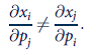Use the Slutsky equation to show that these effects are equal if the individual spends a constant fraction of income on each good regardless of relative prices. (This is a generalization of Problem 6.1.)
EssayExample 6.3 computes the demand functions implied by the three-good CES utility function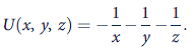a. Use the demand function for x in Equation 6.32 to determine whether x and y or x and z are gross substitutes or gross complements. b. How would you determine whether x and y or x and z are net substitutes or net complements?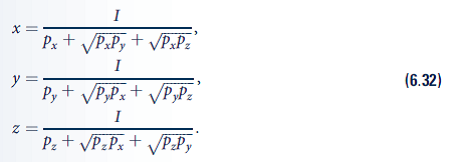EssayConsumer surplus with many goods In Chapter 5, we showed how the welfare costs of changes in a single price can be measured using expenditure functions and compensated demand curves. This problem asks you to generalize this to price changes in two (or many) goods. a. Suppose that an individual consumes n goods and that the prices of two of those goods (say, p 1 and p 2 ) increase. How would you use the expenditure function to measure the compensating variation (CV) for this person of such a price increase? b. A way to show these welfare costs graphically would be to use the compensated demand curves for goods x 1 and x 2 by assuming that one price increased before the other. Illustrate this approach. c. In your answer to part (b), would it matter in which order you considered the price changes? Explain. d. In general, would you think that the CV for a price increase of these two goods would be greater if the goods were net substitutes or net complements? Or would the relationship between the goods have no bearing on the welfare costs?
EssaySeparable utility A utility function is called separable if it can be written as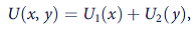where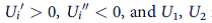need not be the same function. a. What does separability assume about the cross-partial derivative U xy ? Give an intuitive discussion of what word this condition means and in what situations it might be plausible. b. Show that if utility is separable then neither good can be inferior. c. Does the assumption of separability allow you to conclude definitively whether x and y are gross substitutes or gross complements? Explain. d. Use the Cobb-Douglas utility function to show that separability is not invariant with respect to monotonic transformations. Note: Separable functions are examined in more detail in the Extensions to this chapter.Graphing complements Graphing complements is complicated because a complementary relationship between goods (under Hicks' definition) cannot occur with only two goods. Rather, complementarity necessarily involves the demand relationships among three (or more) goods. In his review of complementarity, Samuelson provides a way of illustrating the concept with a two-dimensional indifference curve diagram (see the Suggested Readings). To examine this construction, assume there are three goods that a consumer might choose. The quantities of these are denoted by x 1 , x 2 , and x 3. Now proceed as follows. a. Draw an indifference curve for x 2 and x 3 , holding the quantity of x1 constant at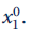. This indifference curve will have the customary convex shape. b. Now draw a second (higher) indifference curve for x 2 , x 3 , holding x1 constant at. For this new indifference curve, show the amount of extra x 2 that would compensate this person for the loss of x 1 ; call this amount j. Similarly, show that amount of extra x 3 that would compensate for the loss of x1 and call this amount k. c. Suppose now that an individual is given both amounts j and k, thereby permitting him or her to move to an even higher x 2 , x 3 indifference curve. Show this move on your graph, and draw this new indifference curve. d. Samuelson now suggests the following definitions: • If the new indifference curve corresponds to the indifference curve when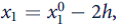, goods 2 and 3 are independent. • If the new indifference curve provides more utility than whengoods 2 and 3 are complements. If the new indifference curve provides less utility than when, goods 2 and 3 are substitutes. Show that these graphical definitions are symmetric. e. Discuss how these graphical definitions correspond to Hicks' more mathematical definitions given in the text. f. Looking at your final graph, do you think that this approach fully explains the types of relationships that might exist between x 2 , and x 3 ,?Details of the analysis suggested in Problems 6.5 and 6.6 were originally worked out by Borcherding and Silberberg (see the Suggested Readings) based on a supposition first proposed by Alchian and Allen. These authors look at how a transaction charge affects the relative demand for two closely substitutable items. Assume that goods x 2 and x 3 are close substitutes and are subject to a transaction charge of t per unit. Suppose also that good 2 is the more expensive of the two goods (i.e., ''good apples'' as opposed to ''cooking apples''). Hence the transaction charge lowers the relative price of the more expensive good [i.e., (p 2 + t)/(p 3 + t) decreases as t increases]. This will increase the relative demand for the expensive good if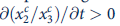(where we use compensated demand functions to eliminate pesky income effects). Borcherding and Silberberg show this result will probably hold using the following steps. a. Use the derivative of a quotient rule to expand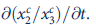. b. Use your result from part (a) together with the fact that, in this problem,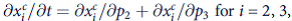to show that the derivative we seek can be written as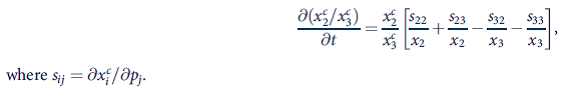c. Rewrite the result from part (b) in terms of compensated price elasticities: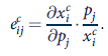d. Use Hicks' third law (Equation 6.26) to show that the term in brackets in parts (b) and (c) can now be written as [(e 22 - e 23 )(1/p 2 - 1/p 3 ) + (e 21 - e 31 )/p 3 ]. e. Develop an intuitive argument about why the expression in part (d) is likely to be positive under the conditions of this problem. Hints: Why is the first product in the brackets positive? Why is the second term in brackets likely to be small? f. Return to Problem 6.6 and provide more complete explanations for these various findings.